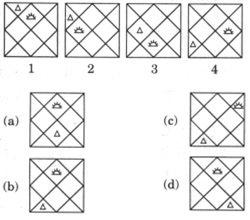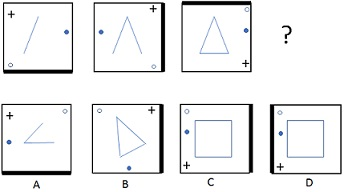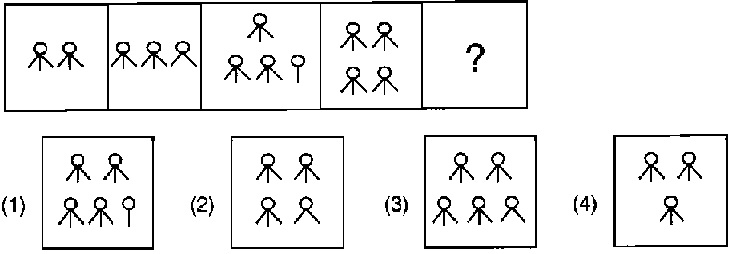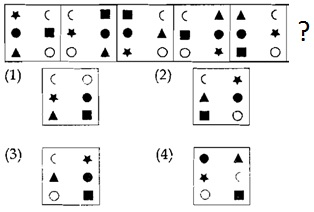# Non Verbal Reasoning Questions and Answers Part 3

9. What will be fifth in the sequence?a. a
b. c
c. b
d. d

Explanation:

The sun moves in anticlockwise direction in the four squares which are in the center.
Also, the triangle is trying to move down, one section at a time in a zigzag manner.

10. What will replace the question mark?a. B
b. A
c. C
d. D

Explanation:

Since one line increases every time, next one must have a square.
+ sign moves around corners in clockwise direction.
Blank circle moves around corners in anti-clockwise direction.
Filled circle moves left and right alternately.

11. What will be next in the sequence out of the given answer figures?a. 3
b. 4
c. 1
d. 2

Explanation:

A figure consists of three lines (do not consider the circles). Two lines get added every time. If a new figure is to be started, a circle gets added automatically like in diagram number 3.

12. Which of the options will replace the question mark?a. 1
b. 4
c. 3
d. 2